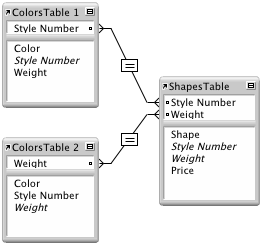# Choosing the evaluation context for a calculation field

Context is the table in the relationships graph from which a relationship is evaluated. When you define a calculation in a source table, and the table has more than one occurrence in the relationships graph, you must specify the context from which you want the calculation to evaluate.

Each context choice represents a different table in the relationships graph, and a different perspective into your data; when you choose the context for a calculation, you tell FileMaker Pro to evaluate the calculation starting from that table occurrence. Because each context choice represents a different association of related tables and fields, choosing the evaluation context for your calculation is critical to getting the results you expect.

## An example of how context changes the results of a calculation

You have a database with two source tables named ColorsSourceTable and ShapesSourceTable. The tables have these properties:

 Source table name Table occurrences in relationships graph Field names ColorsSourceTable ColorsTable 1, ColorsTable 2 Color Style Number Weight ShapesSourceTable ShapesTable Shape Style Number Weight Price

The tables are related as follows:There are two separate relationships:

• In the first, ColorsTable 1 and ShapesTable are related using the Style Number fields.
• In the second, ColorsTable 2 and ShapesTable are related using the Weight fields.

Because ColorsTable 1 and ColorsTable 2 share the same source table, a calculation defined in ColorsSourceTable can be evaluated from the context of either the ColorsTable 1 table occurrence or the ColorsTable 2 table occurrence.

To see how this works, consider the effects of context on two calculation fields in the table ColorsSourceTable. Each calculation field uses the same formula to determine the sum of prices in the table ShapesSourceTable; the only change is the evaluation context. ShapesSourceTable has three records with these values:

 Shape Style Number Weight Price circle 100 5 \$2.00 square 100 2 \$6.00 triangle 200 2 \$9.00

A single record in the ColorsSourceTable has these values:

 Color Style Number Weight red 100 2

Because the relationships use different match fields, each relationship returns a different set of related records.

## Calculation result 1

The relationship between ColorsTable 1 and ShapesTable, which uses Style Number as the match field, returns these two records:

 Shape Style Number Weight Price circle 100 5 \$2.00 square 100 2 \$6.00

A calculation field defined in the ColorsSourceTable with the formula `Sum(ShapesTable::Price)` that is set to evaluate from the context of the table ColorsTable 1 returns the value \$8.00.

## Calculation result 2

The relationship between ColorsTable 2 and ShapesTable, which uses Weight as the match field, returns these two records:

 Shape Style Number Weight Price square 100 2 \$6.00 triangle 200 2 \$9.00

A second calculation field defined in the ColorsSourceTable with the same formula, `Sum(ShapesTable::Price)`, but set to evaluate from the context of the table ColorsTable 2 returns the value \$15.00.

## Notes

• When constructing a calculation formula, evaluation context determines which tables appear as related and unrelated in the Specify Calculation dialog box.
• A calculation formula can use global fields from unrelated tables. These are the only fields that can be used from unrelated tables.